# Lines

A line is a one-dimensional figure, which has length but no width. A line is made of a set of points which is extended in opposite directions infinitely. It is determined by two points in a two-dimensional plane. The two points which lie on the same line are said to be collinear points.

In geometry, there are different types of lines such as horizontal and vertical lines, parallel and perpendicular lines. These lines play an important role in the construction of different types of polygons. For example, a square is made by four lines of the same lengths, whereas a triangle is made by joining three lines end to end.

## What is a Line?

• A line can be defined as a straight set of points that extend in opposite directions
• It has no ends in both directions(infinite)
• It has no thickness
• it is one-dimensional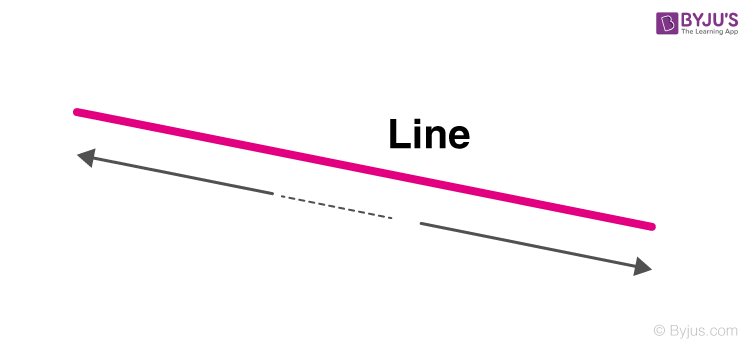### Points, Lines and Angles

Points, lines and angles are the basics of geometry which collectively define the shapes of an object.

An example of a combination of points, lines and angles is a rectangle which has four vertices defined by a point, four sides shown by lines and four angles equal to 90 degrees.

Similarly, we can define other shapes such as rhombus, parallelogram, square, kite, cube, cuboid, etc using these three primary figures.

## What is a Line segment?

• A line segment is part of a line which has defined endpoints
• It has a beginning point and an ending point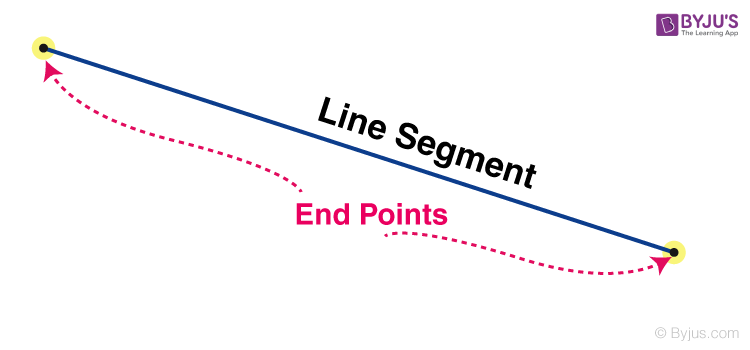## What is a Ray?

• A ray is a part of a line that has one endpoint (i.e. starting point) and it extends in one direction endlessly.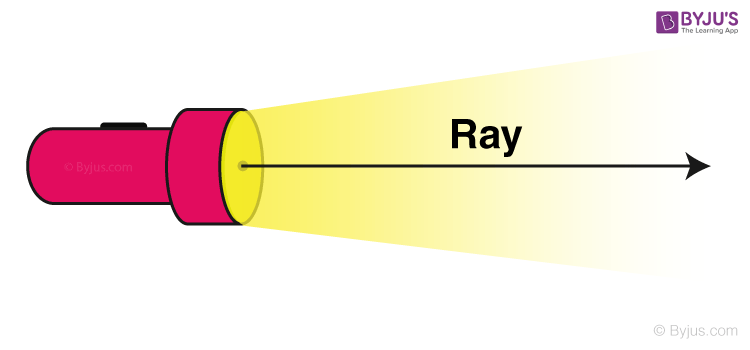## Types of Line

In Geometry, there are basically four types of lines. They are:

### Horizontal Lines

When a line moves from left to right in a straight direction, it is a horizontal line.### Vertical Lines

When a line runs from top to bottom in a straight direction, it is a vertical line.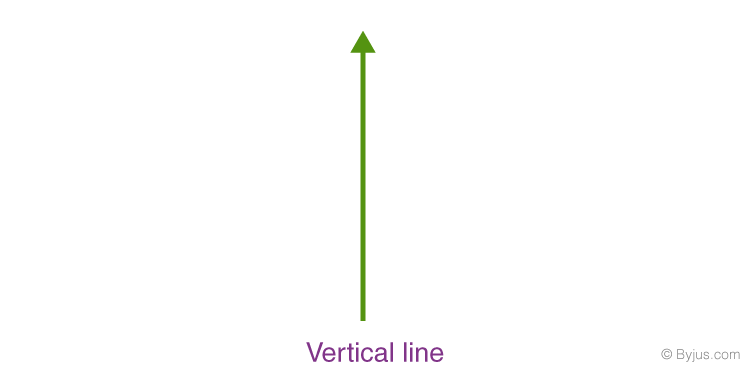### Parallel Lines

When two straight lines don’t meet or intersect at any point, even at infinity, then they are parallel to each other.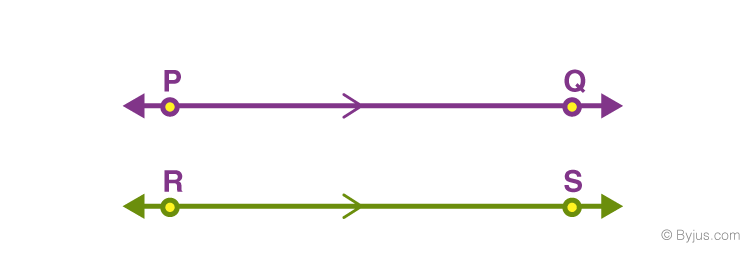Suppose two lines PQ and RS are parallel then it is represented as PQ||RS.

### Perpendicular Lines

When two lines meet or intersect at an angle of 90 degrees or at a right angle, then they are perpendicular to each other.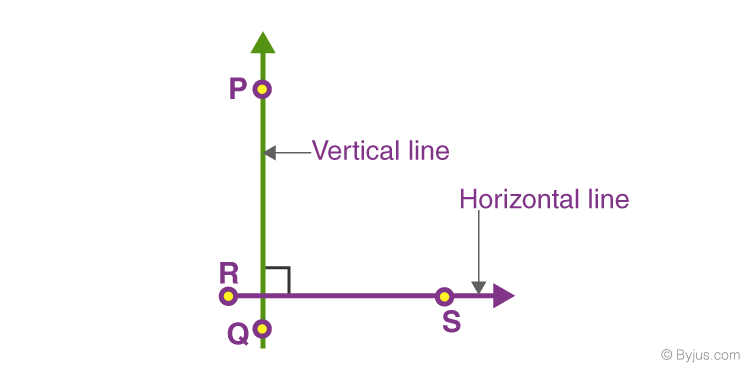If PQ and RS are two lines which are perpendicular to each other, then it is represented as PQ ⊥ RS.

### Some Other types of Lines in Maths

#### 1.Tangent lines

The Tangent is a straight line which just touches the curve at a given point. The normal is a straight line which is perpendicular to the tangent. To calculate the equations of these lines, we shall make use of the fact that the equation of a straight line passing through the point with coordinates (x1, y1) and having gradient m is given by

$$\begin{array}{l}\frac{y-y_{1}}{x-x_{1}}=1\end{array}$$

We also make use of the fact that if two lines with gradients m1 and m2 respectively are perpendicular, then m1m2 = −1.

Example: Suppose we wish to find points on the curve y(x) given by x3 – 6x2 + x + 3  where the tangents are parallel to the line y = x + 5.

Solution: If the tangents have to be parallel to the line then they must have the same gradient. The standard equation for a straight line is y = mx + c, where m is the gradient. So what we gain from looking at this standard equation and comparing it with the straight line y = x + 5 is that the gradient, m, is equal to 1. Thus, the gradients of the tangents we are trying to find must also have gradient 1.

We know that if we differentiate y(x) we will obtain an expression for the gradients of the tangents to y(x) and we can set this equal to 1. Differentiating and making it equal to 1 we find:

$$\begin{array}{l}\frac{dy}{dx}=3x^{2}-12x+1=1\end{array}$$

from which

$$\begin{array}{l}3x^{2} – 12x = 0\end{array}$$

This is a quadratic equation which we can solve by factorisation.

$$\begin{array}{l}3x^{2} – 12x = 0\end{array}$$

$$\begin{array}{l}3x (x-4) = 0\end{array}$$

$$\begin{array}{l}\Rightarrow 3x = 0 \;\; or \;\; (x-4) = 0\end{array}$$

$$\begin{array}{l}\Rightarrow x = 0 \; or \ x = 4\end{array}$$

Now having found these two values of x we can calculate the corresponding y coordinates. We do this from the equation of the curve:

$$\begin{array}{l}y = x^{3} – 6x^{2} + x + 3 \end{array}$$

when x = 0:

$$\begin{array}{l} y =(0)^{3} – 6(0)^{2} + 0 + 3 = 3\end{array}$$

when x = 4:

$$\begin{array}{l}y =(4)^{3} – 6(4)^{2} + 4 + 3= -25 \end{array}$$

So the two points are (0, 3) and (4, −25)

These are the two points where the gradients of the tangent are equal to 1, and so where the tangents are parallel to the line that we started out with, i.e. y = x + 5.

#### 2. Secant lines

A line in the plane is a secant line to a circle if it meets the circle in exactly two points. It is also equivalent to the average rate of change, or simply the slope between two points. The average rate of change of a function between two points and the slope between two points are the same thing.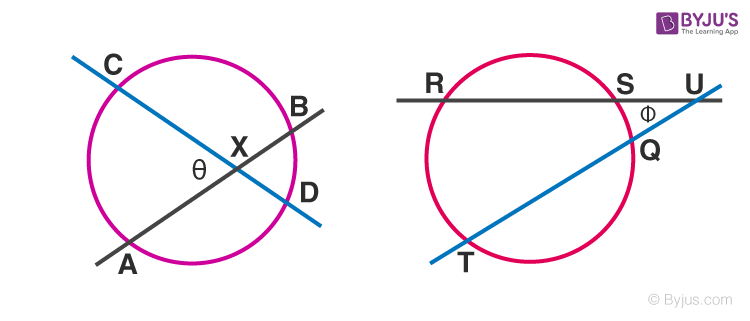In the left figure above,

θ = (1/2)(arc A C + arc B D),

while in the right figure,

Φ = (1/2)(arc R T – arc S Q),

Where arc AB denotes the angular measure of the arc AB.

## Frequently Asked Questions – FAQs

Q1

### How to define a line?

A line is a figure in geometry, which has only length and no width in a two-dimensional plane and extends infinitely in opposite directions.
Q2

### What are the types of lines?

In geometry there are four types of lines:
Horizontal Line
Vertical lines
Parallel lines
Perpendicular lines
Q3

### What is a vertical line?

A vertical line is a line drawn from the bottom to the top in a straight direction.
Q4

### What is a horizontal line?

A horizontal line is a line drawn from left to right in a straight direction.
Q5

### What is a line segment?

A line segment is a part of line which has two fixed endpoints.